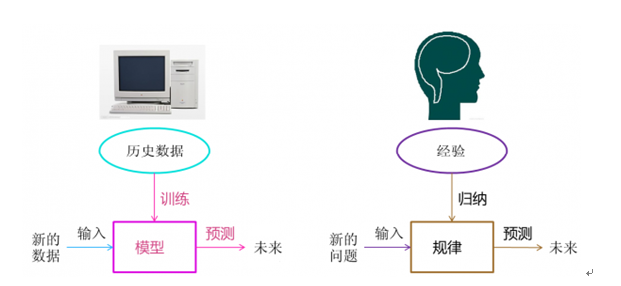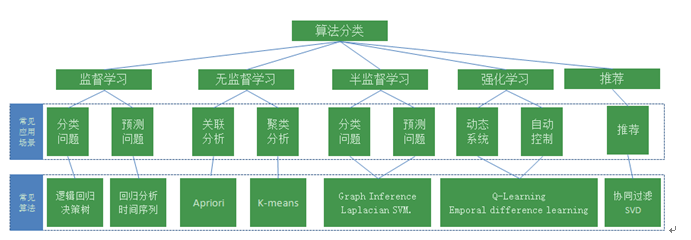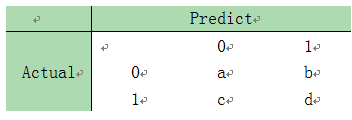## 互联网金融时代下机器学习与大数据风控系统

1 评论 3.3万 浏览 129 收藏 15 分钟### 什么是机器学习？A computer program is said to learn from experience E with respect to some task T and some performance measure P, if its performance on T, as measured by P, improves with experience E.### 机器学习算法的类型### 机器学习在互联网金融行业中的应用

F(x)=F_0+β_1 T_1 (x)+β_2 T_2 (x)+⋯+β_m T_m (x)

GBDT在被提出之初就和SVM一起被认为是泛化能力（generalization）较强的算法。近些年更因被广泛应用于搜索排序以及推荐中而引起大家的关注，如Yahoo, Ebay等大型互联网公司就采用过GBDT进行搜索排序。在国内，我在携程工作时就曾应用GBDT算法对客人进行酒店noshow和延住的预测，为公司每年带来千万的收入，该项目是携程技术驱动业务发展的典型代表。

GBDT作为一种boosting算法，自然包含了boosting的思想，即将一系列弱分类器组合起来构成一个强分类器。它不要求每个分类器都学到太多的东西，只要求每个分类器都学一点点知识，然后将这些学到的知识累加起来构成一个强大的模型。

### 分类模型的性能评估

1）Confusion Matrix1. a是正确预测到的负例的数量，True Negative(TN)
2. b是把负例预测成正例的数量，False Positive (FP)
3. c是把正例预测成负例的数量，False Negative(FN)
4. d是正确预测到的正例的数量，True Positive(TP)
5. a+b是实际上为负例的数量，Actual Negative
6. c+d是实价上为正例的数量，Actual Positive
7. a+c是预测成负例的数量，Predicted Negative
8. b+d是预测成正例的数量，Predicted Positive

2）Accuracy（准确分类率）

Accuracy=(true positive and true negative)/total=(a+d)/(a+b+c+d)

3）Error Rate（误分类率）

Error Rate=(false positive and false negative)/total=(c+b)/(a+b+c+d)

4）Recall（正例覆盖率）

recall也称为sensitivity,在机器学习中称recall较多，而sensitivity是生物统计中的常用叫法

Recall=(true positive )/(actual positive)=d/(c+d)

5）Performance也称为Precision（正例的命中率简称命中率）

Performance=(true positive )/(predicted positive)=d/(b+d)

6）Specificity（负例覆盖率）

Specificity=(true negative )/(actual negative)=a/(a+b)

7）Negative predicted value (负例命中率)

Negative predicted value=(true negative )/(predicted negative)=a/(a+c)

8）ROC

ROC曲线就是不同的阈值下，Sensitivity和1-Specificity的组合,ROC曲线是根据与45度线的偏离来判断模型好坏。9）AUC与GINI

AUC=ROC曲线下的面积

GINI=2AUC-1

10）K-S1. 写的挺好。

回复